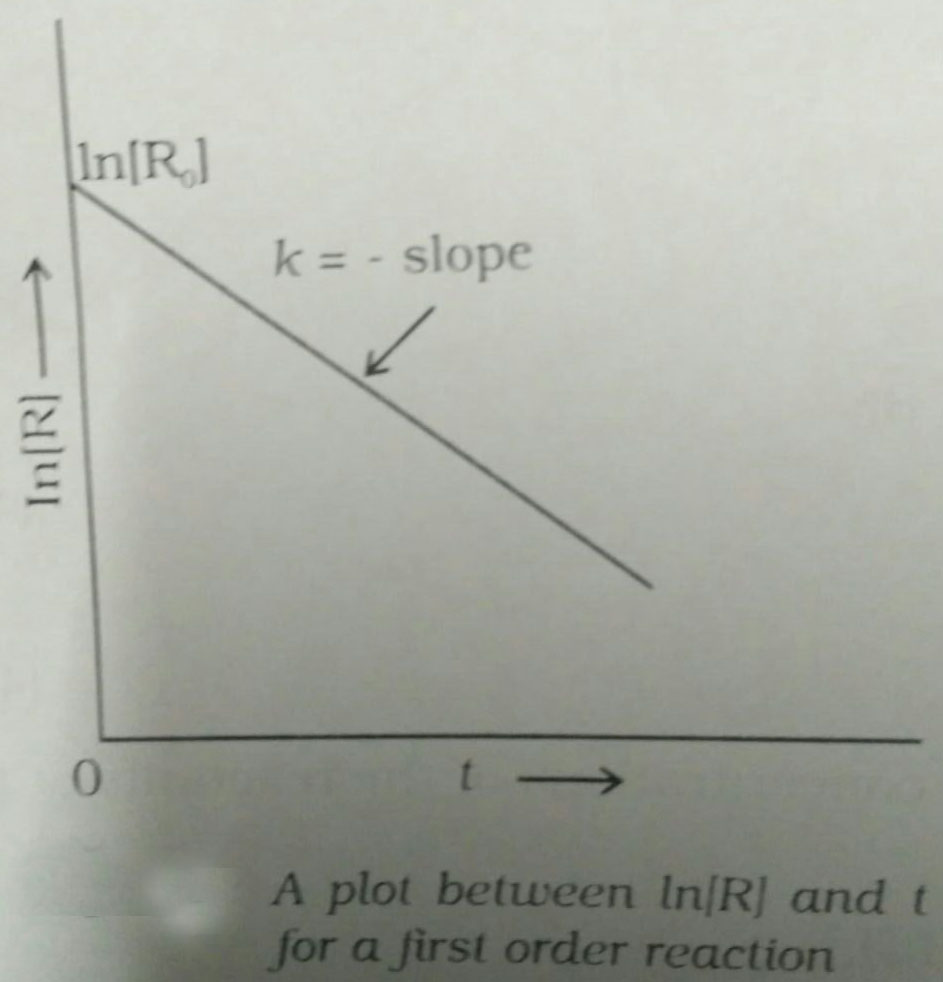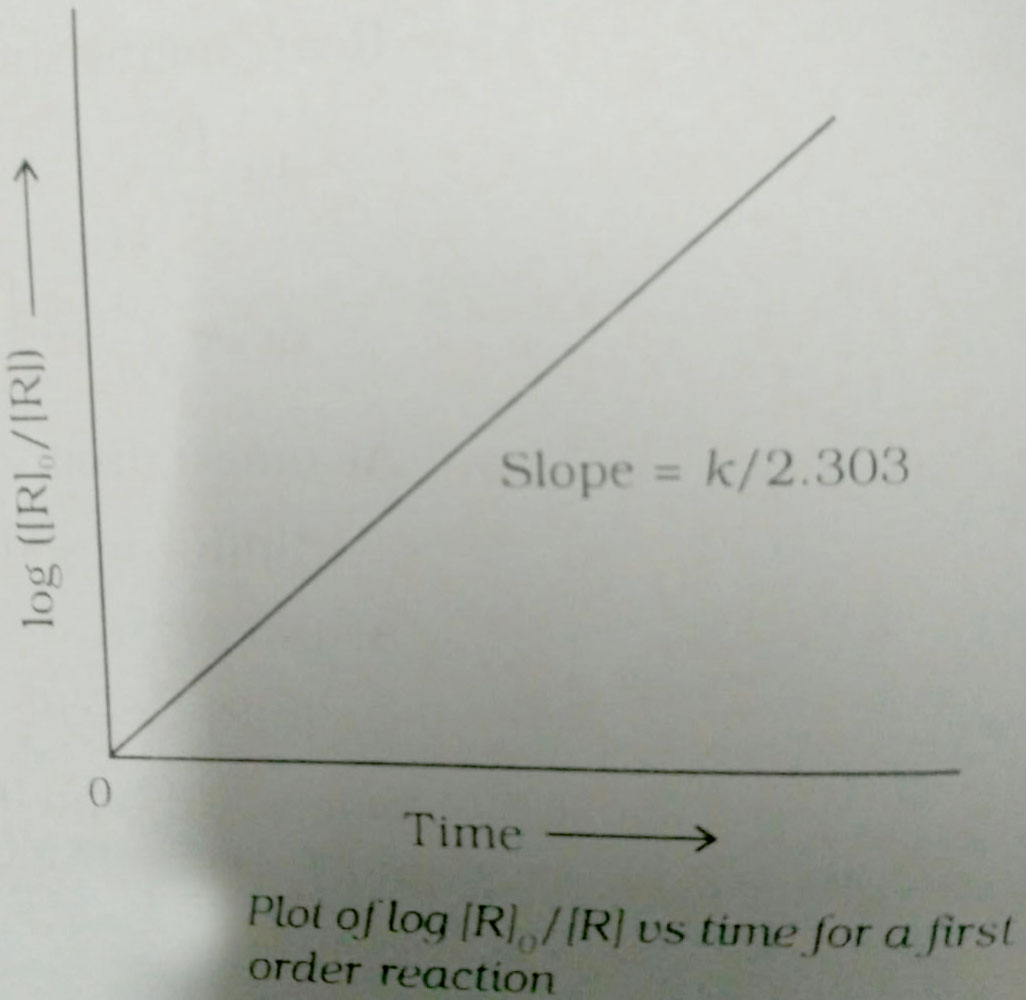Q

# I need help with The half-­life of a radioisotope is four hours. If the initial mass of the isotope was 200 g, the mass remaining after 24 hours undecayed is

The half-­life of a radioisotope is four hours. If the initial mass of the isotope was 200 g, the mass remaining after 24 hours undecayed is

• Option 1)

1.042 g

• Option 2)

2.084 g

• Option 3)

3.125 g

• Option 4)

4.167 g.

142 Views

As learnt in the concept

First Order Reaction -

The rate of the reaction is proportional to the first power of the concentration of the reaction- wherein

Formula:

R            P

a                 0

a-x             x

[differentiate rate law]

Unit of

Option 1)

1.042 g

This option is incorrect.

Option 2)

2.084 g

This option is incorrect.

Option 3)

3.125 g

This option is correct.

Option 4)

4.167 g.

This option is incorrect.

Exams
Articles
Questions